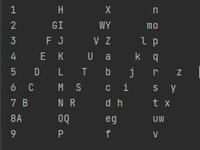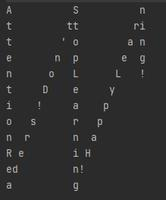Related Articles

# Program to print a string in vertical zigzag manner

• Difficulty Level : Hard
• Last Updated : 06 Jul, 2021

Given a string, S of size N, and a number of rows R, the task is to print the given string in a vertical zigzag fashion with respect to the given number of rows as shown in the examples.

Examples:

Input: S = “123456789ABCDEFGHIJKLMNOPQRSTUVWXYZabcdefghijklmnopqrstuvwxyz”, R = 9
Output:Input: S = “AttentionReaders!Don’tStopLearning!HappyLearning!”, R = 12
Output:Approach: In order to print the characters line by line, the idea is to find the interval between the major columns and step value for in-between columns for printing the spaces until the last character of the string is reached. Follow the steps below to solve this problem:

• Initialize a variable interval as 2*R-2 to store the gap between the major columns.
• Iterate in the range [0, R-1] using the variable i
• Initialize a variable step as interval-2*i to store step values for each row.
• Iterate in the range [i, N-1] using the variable j, incrementing j by interval in each iteration,
• Print the character, S[j].
• If the value of step lies in the range [1, interval-1] and step+j<N, then print (interval-R-i) number of spaces, then print s[j+step] and finally print (i-1) spaces.
• Else print (interval-R) number of spaces.
• Print newline after each iteration of the outer loop.

Below is the implementation of the above approach:

## C++

 `// C++ program for the above approach``#include ``using` `namespace` `std;` `// Function to print any string``// in zigzag fashion``void` `zigzag(string s, ``int` `rows)``{``    ``// Store the gap between the major columns``    ``int` `interval = 2 * rows - 2;` `    ``// Traverse through rows``    ``for` `(``int` `i = 0; i < rows; i++) {` `        ``// Store the step value for each row``        ``int` `step = interval - 2 * i;` `        ``// Iterate in the range [1, N-1]``        ``for` `(``int` `j = i; j < s.length(); j = j + interval) {` `            ``// Print the character``            ``cout << s[j];``            ``if` `(step > 0 && step < interval``                ``&& step + j < s.length()) {` `                ``// Print the spaces before character``                ``// s[j+step]``                ``for` `(``int` `k = 0; k < (interval - rows - i);``                     ``k++)``                    ``cout << ``" "``;` `                ``// Print the character``                ``cout << s[j + step];` `                ``// Print the spaces after character``                ``// after s[j+step]``                ``for` `(``int` `k = 0; k < i - 1; k++)``                    ``cout << ``" "``;``            ``}``            ``else` `{` `                ``// Print the spaces for first and last rows``                ``for` `(``int` `k = 0; k < (interval - rows); k++)``                    ``cout << ``" "``;``            ``}``        ``}``        ``cout << endl;``    ``}``}` `// Driver Code``int` `main()``{``    ``// Given Input``    ``string s = ``"123456789ABCDEFGHIJKLMNOPQRSTUVWXYZabcdefgh"``               ``"ijklmnopqrstuvwxyz"``;``    ``int` `rows = 9;` `    ``// Function Call``    ``zigzag(s, rows);``}`

## Java

 `// Java program for the above approach` `public` `class` `GFG{` `// Function to print any string``// in zigzag fashion``static` `void` `zigzag(String s, ``int` `rows)``{``    ` `    ``// Store the gap between the major columns``    ``int` `interval = ``2` `* rows - ``2``;` `    ``// Traverse through rows``    ``for``(``int` `i = ``0``; i < rows; i++)``    ``{``        ` `        ``// Store the step value for each row``        ``int` `step = interval - ``2` `* i;` `        ``// Iterate in the range [1, N-1]``        ``for``(``int` `j = i; j < s.length(); j = j + interval)``        ``{``            ` `            ``// Print the character``            ``System.out.print(s.charAt(j));``            ``if` `(step > ``0` `&& step < interval &&``                ``step + j < s.length())``            ``{``                ` `                ``// Print the spaces before character``                ``// s[j+step]``                ``for``(``int` `k = ``0``; k < (interval - rows - i); k++)``                    ``System.out.print(``" "``);` `                ``// Print the character``                ``System.out.print(s.charAt(j + step));` `                ``// Print the spaces after character``                ``// after s[j+step]``                ``for``(``int` `k = ``0``; k < i - ``1``; k++)``                    ``System.out.print(``" "``);``            ``}``            ``else``            ``{``                ` `                ``// Print the spaces for first and last rows``                ``for``(``int` `k = ``0``; k < (interval - rows); k++)``                    ``System.out.print(``" "``);``            ``}``        ``}``        ``System.out.println();``    ``}``}` `// Driver Code``public` `static` `void` `main(String args[])``{``    ` `    ``// Given Input``    ``String s = ``"123456789ABCDEFGHIJKLM"` `+``               ``"NOPQRSTUVWXYZabcdefghi"` `+``               ``"jklmnopqrstuvwxyz"``;``    ``int` `rows = ``9``;` `    ``// Function Call``    ``zigzag(s, rows);``}``}` `// This code is contributed by SoumikMondal`

## Python3

 `# Python3 program for the above approach` `# Function to print any string``# in zigzag fashion``def` `zigzag(s, rows):``    ` `    ``# Store the gap between the major columns``    ``interval ``=` `2` `*` `rows ``-` `2` `    ``# Traverse through rows``    ``for` `i ``in` `range``(rows):``        ` `        ``# Store the step value for each row``        ``step ``=` `interval ``-` `2` `*` `i` `        ``# Iterate in the range [1, N-1]``        ``for` `j ``in` `range``(i, ``len``(s), interval):``            ` `            ``# Print the character``            ``print``(s[j], end ``=` `"")``            ` `            ``if` `(step > ``0` `and` `step < interval ``and``                         ``step ``+` `j < ``len``(s)):` `                ``# Print the spaces before character``                ``# s[j+step]``                ``for` `k ``in` `range``((interval ``-` `rows ``-` `i)):``                    ``print``(end ``=` `" "``)` `                ``# Print the character``                ``print``(s[j ``+` `step], end ``=` `"")` `                ``# Print the spaces after character``                ``# after s[j+step]``                ``for` `k ``in` `range``(i ``-` `1``):``                    ``print``(end ``=` `" "``)``            ``else``:` `                ``# Print the spaces for first and``                ``# last rows``                ``for` `k ``in` `range``(interval ``-` `rows):``                    ``print``(end ``=` `" "``)``                    ` `        ``print``()` `# Driver Code``if` `__name__ ``=``=` `'__main__'``:``    ` `    ``# Given Input``    ``s ``=` `"123456789ABCDEFGHIJKL"``\``        ``"MNOPQRSTUVWXYZabcdefghi"``\``        ``"jklmnopqrstuvwxyz"``    ``rows ``=` `9` `    ``# Function Call``    ``zigzag(s, rows)` `# This code is contributed by mohit kumar 29`

## C#

 `// C# program for the above approach``using` `System;``using` `System.Collections.Generic;` `class` `GFG{` `// Function to print any string``// in zigzag fashion``static` `void` `zigzag(``string` `s, ``int` `rows)``{``    ` `    ``// Store the gap between the major columns``    ``int` `interval = 2 * rows - 2;` `    ``// Traverse through rows``    ``for``(``int` `i = 0; i < rows; i++)``    ``{``        ` `        ``// Store the step value for each row``        ``int` `step = interval - 2 * i;` `        ``// Iterate in the range [1, N-1]``        ``for``(``int` `j = i; j < s.Length; j = j + interval)``        ``{``            ` `            ``// Print the character``            ``Console.Write(s[j]);``            ``if` `(step > 0 && step < interval &&``                ``step + j < s.Length)``            ``{``                ` `                ``// Print the spaces before character``                ``// s[j+step]``                ``for``(``int` `k = 0; k < (interval - rows - i); k++)``                    ``Console.Write(``" "``);` `                ``// Print the character``                ``Console.Write(s[j + step]);` `                ``// Print the spaces after character``                ``// after s[j+step]``                ``for``(``int` `k = 0; k < i - 1; k++)``                    ``Console.Write(``" "``);``            ``}``            ``else``            ``{``                ` `                ``// Print the spaces for first and last rows``                ``for``(``int` `k = 0; k < (interval - rows); k++)``                    ``Console.Write(``" "``);``            ``}``        ``}``        ``Console.WriteLine();``    ``}``}` `// Driver Code``public` `static` `void` `Main()``{``    ` `    ``// Given Input``    ``string` `s = ``"123456789ABCDEFGHIJKLM"` `+``               ``"NOPQRSTUVWXYZabcdefghi"` `+``               ``"jklmnopqrstuvwxyz"``;``    ``int` `rows = 9;` `    ``// Function Call``    ``zigzag(s, rows);``}``}` `// This code is contributed by SURENDRA_GANGWAR`

## Javascript

 ``
Output
```1       H       X       n
2      GI      WY      mo
3     F J     V Z     l p
4    E  K    U  a    k  q
5   D   L   T   b   j   r   z
6  C    M  S    c  i    s  y
7 B     N R     d h     t x
8A      OQ      eg      uw
9       P       f       v       ```

Time Complexity: O(R2*N)
Auxiliary Space: O(1)

Attention reader! Don’t stop learning now. Get hold of all the important DSA concepts with the DSA Self Paced Course at a student-friendly price and become industry ready.  To complete your preparation from learning a language to DS Algo and many more,  please refer Complete Interview Preparation Course.

In case you wish to attend live classes with experts, please refer DSA Live Classes for Working Professionals and Competitive Programming Live for Students.

My Personal Notes arrow_drop_up